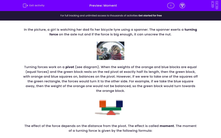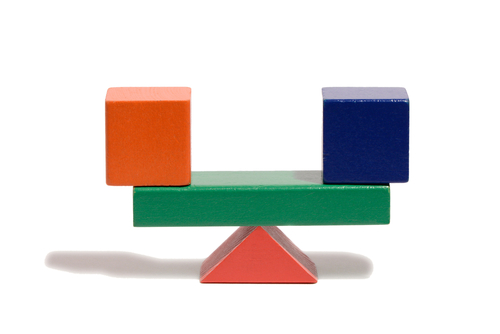# Calculate Moments and Explain Why Things Balance

In this worksheet, students will be introduced to the principle of moments and understand what turning forces are.Key stage:  KS 3

Curriculum topic:   Physics: Motion and Forces

Curriculum subtopic:   Motion and Forces

Difficulty level:#### Worksheet Overview

In the picture, a girl is watching her dad fix her bicycle tyre using a spanner. The spanner exerts a turning force on the axle nut and if the force is big enough, it can unscrew the nut.Turning forces work on a pivot (see diagram). When the weights of the orange and blue blocks are equal (equal forces) and the green block rests on the red pivot at exactly half its length, then the green block, with orange and blue squares on, balances on the pivot. However, if we were to take one of the squares off the green rectangle, the forces would turn it to the other side. For example, if we take the blue square away, then the weight of the orange one would not be balanced, so the green block would turn towards the orange block.The effect of the force depends on the distance from the pivot. The effect is called moment. The moment of a turning force is given by the following formula:

moment  =  force applied  ×  distance from a pivot

Moment is measured in newton centimetres (Ncm) or newton metres (Nm). In order to increase the effect of a turning force, one can either increase the force itself by pulling or pushing harder, or can increase the distance from the pivot.

The cartoon shows a see-saw that is balanced because of the way a family have sat on it.The total moment of the people sitting on the right side is equal to the total moment of the people sitting on the left side:

total clockwise moment  =  total anticlockwise moment

### What is EdPlace?

We're your National Curriculum aligned online education content provider helping each child succeed in English, maths and science from year 1 to GCSE. With an EdPlace account you’ll be able to track and measure progress, helping each child achieve their best. We build confidence and attainment by personalising each child’s learning at a level that suits them.

Get started